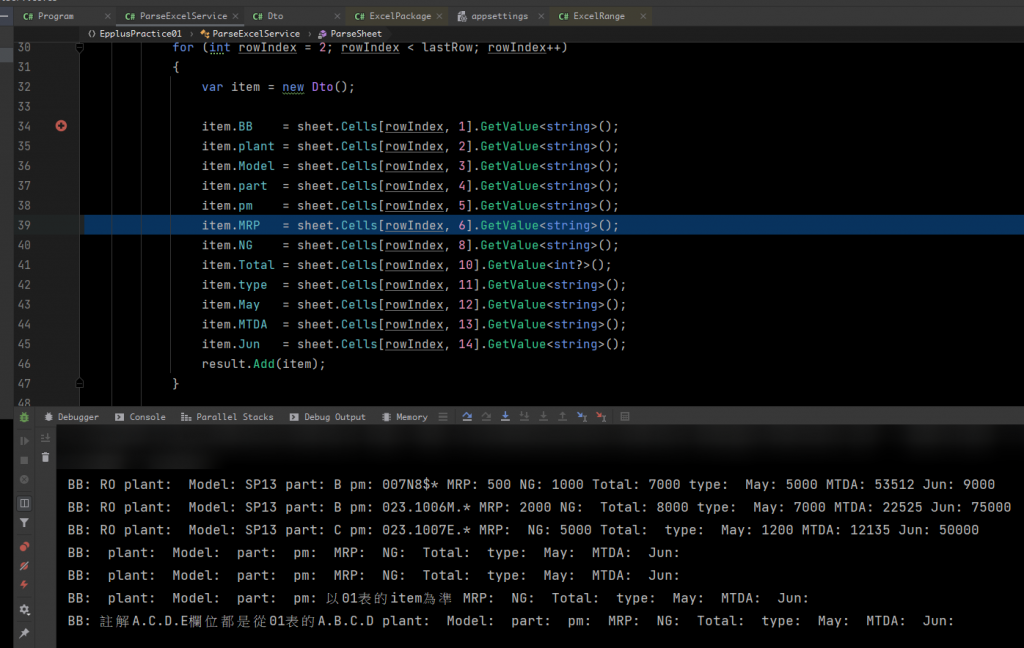#0

## C# Excel 處理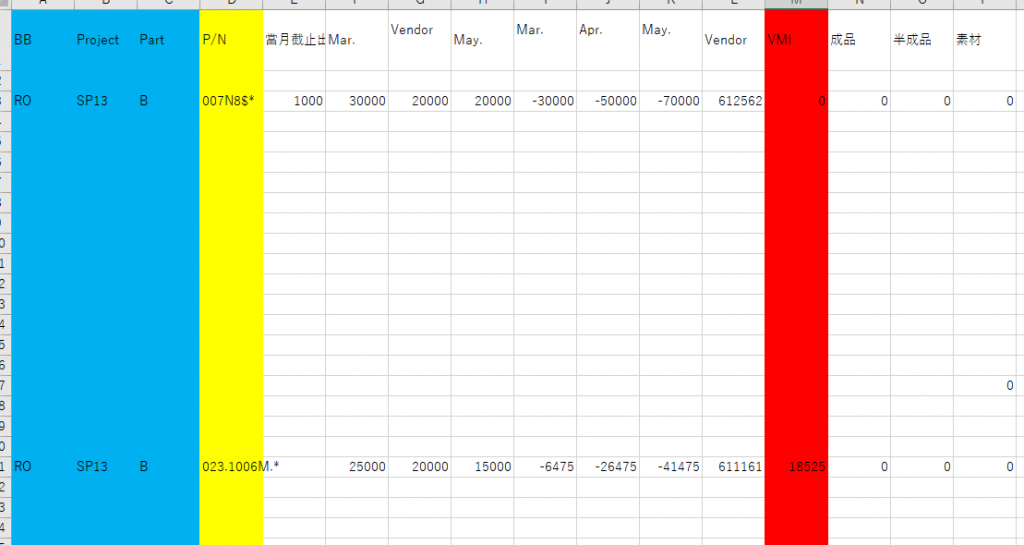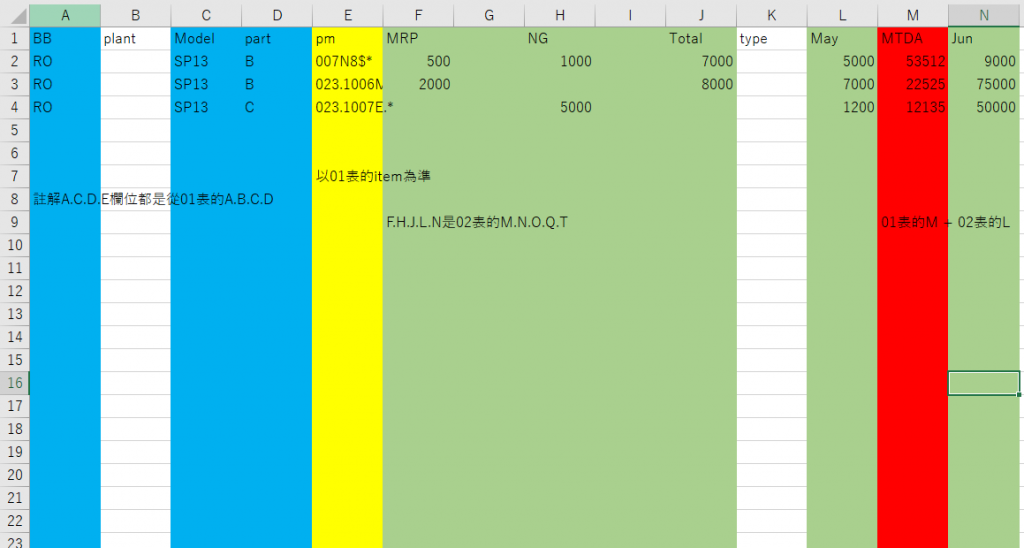``````  var dataOrigin = new List<DataOrigin>();
for(int r = 1; r <= totalRows; r++ )
{
{ item = sh.Cells[r, 1].Value.ToString(),
Apr = sh.Cells[r, 2].Value.ToString(),
May = sh.Cells[r, 3].Value.ToString(),
Jun = sh.Cells[r, 4].Value.ToString()
});
}

``````

### 2 個回答

0
``````item = sh.Cells[r, 1].Value == NULL ? "" : sh.Cells[r, 1].Value.ToString().ToString()
``````

``````  for (int r = 3; r <= totalColums; r++)
{
{
project = sh1.Cells[r, 1].Value?.ToString(),
part = sh1.Cells[r, 2].Value?.ToString(),
im = sh1.Cells[r, 3].Value?.ToString(),
VM = sh1.Cells[r, 4].Value?.ToString()
});
}
``````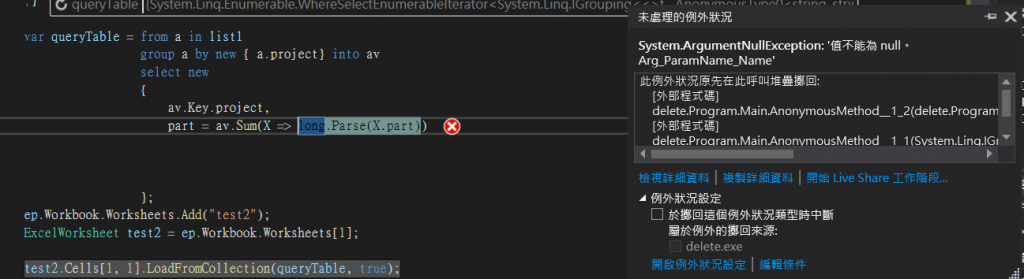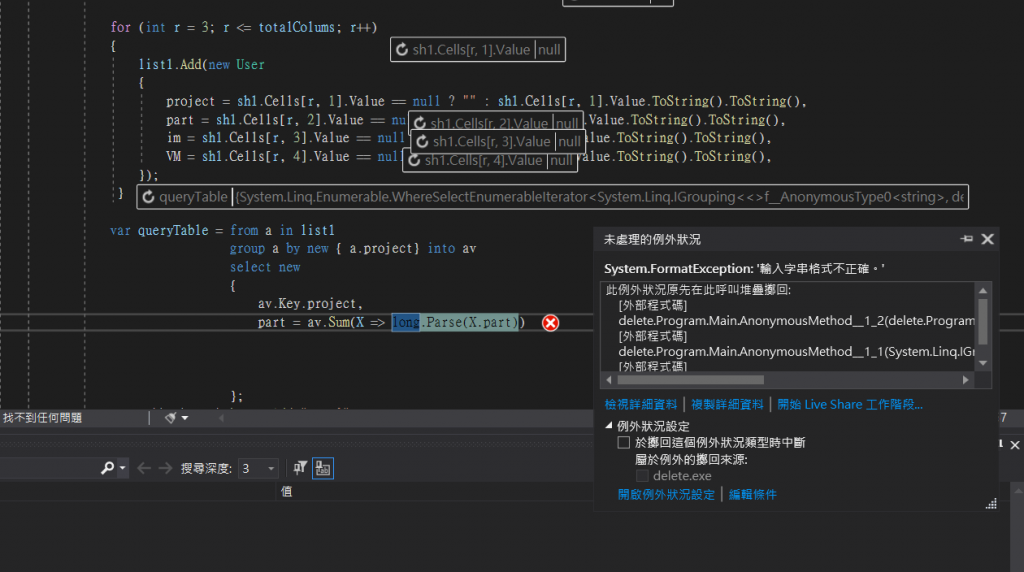``````item = sh.Cells[r, 1].Value == NULL ? 0 : Int64.Parse(sh.Cells[r, 1].Value)
``````0

Github 範例